TitleCollege Algebra
Answer/Discussion to Practice Problems
Tutorial
46: Logarithmic EquationsAnswer/Discussion to 1a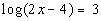This is already done for us in this problem.

 Step 2: Use the definition of logarithms to write in exponential form.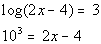*Rewrite in exponential form *Base = 10 and exponent = 3

 Remember that when there is no base written on a log, that means it is the common log, or log base 10.  If you need a review on common logs feel free to go to Tutorial 43: Logarithmic Functions.

 Step 3: Solve for x.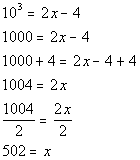*10 cubed is 1000 *Solve for xAnswer/Discussion to 1b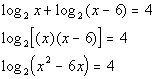*Use the product rule to write as one log *one log is isolated

 Step 2: Use the definition of logarithms to write in exponential form.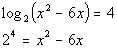*Rewrite in exponential form *Base = 2 and exponent = 4

 Step 3: Solve for x.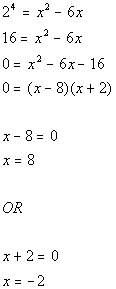*2 to the 4th power is 16 *Solve for x *Set 1st factor = 0          *Set 2nd factor = 0

 Since -2 would create a negative number inside both logs in this problem and we CANNOT take the log of a negative number, we will have to throw out x = -2 as one of our solutions.   Final answer: x = 8.Answer/Discussion to 1c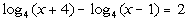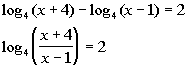*Use the quotient rule to write as one log *one log is isolated

 Step 2: Use the definition of logarithms to write in exponential form.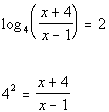*Rewrite in exponential form *Base = 4 and exponent = 2

 Step 3: Solve for x.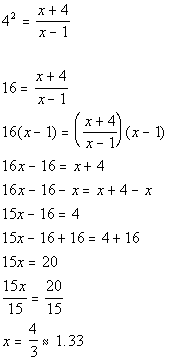*4 squared is 16 *Solve for x   *Multiply both sides by LCD of x - 1

 The equation that we had to solve in step 3 had a rational expression in it.  If you need a review on solving equations with rational expressions feel free to go to Tutorial 15: Equations with Rational Expressions.Answer/Discussion to 1d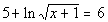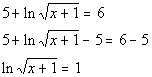*Inverse of add 5 is sub. 5 *one log is isolated

 Step 2: Use the definition of logarithms to write in exponential form.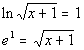*Rewrite in exponential form *Base = e and exponent = 1

 Remember that when you have ln, that means it is the natural log, or log base e.  If you need a review on natural logs feel free to go to Tutorial 43: Logarithmic Functions.

 Step 3: Solve for x.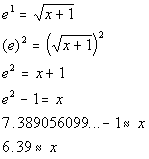*Square both sides to get rid of the radical *Solve for x   *Use the calculator to find e squared

 The equation that we had to solve in step 3 had a square root in it.  If you need a review on solving equations with radicals feel free to go to Tutorial 19: Radical Equations and Equations Involving Rational Exponents.

Last revised on March 23, 2011 by Kim Seward.
All contents copyright (C) 2002 - 2011, WTAMU and Kim Seward. All rights reserved.